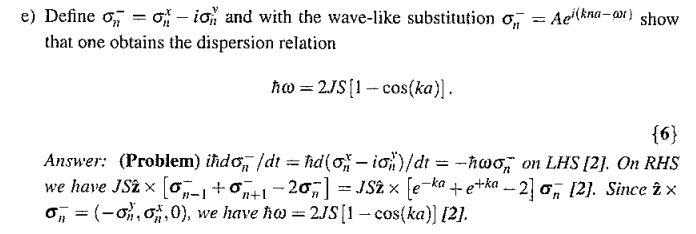# Heisenburg Ferromagnetic Model

## Homework Statement

There are several parts to this question, however I could complete these parts. It is just an equation used in the prior part to the question that is need to solve this:

If we define \begin{equation} \sigma_{n}^{-}=\sigma_{n}^{x}+i\sigma_{n}^{y} \end{equation} and with the wavelike substitution \begin{equation} \sigma_{n}^{-}=Ae^{i(kna-wt)} \end{equation} show that one obtains the dispersion relation: \begin{equation} \hbar\omega=2JS[1-cos(ka)] \end{equation}

## Homework Equations

The substitution referred to is into this formula:

\begin{equation} \hbar \frac{d\mathbf{\sigma}_{n}}{dt}=JS\hat{z}\times (\sigma_{n-1}-2\sigma_{n}+\sigma_{n+1}) \end{equation} and we also know: \begin{equation} \sigma_{n}=(\sigma_{n}^{x},\sigma_{n}^{y},0) \end{equation}

## The Attempt at a Solution

I thought it would simply be that we substitute in the \begin{equation} \sigma_{n}^{-}=Ae^{i(kna-wt)} \end{equation} into the equation and solve. However, that wouldn't make sense to me as the \begin{equation} \sigma_{n}^{-} \end{equation} value does not seem to be defined as a vector but the right hand side of the equation is. Even so when we do this the differential on the LHS would return a factor of 'i' and I'm not sure where that would disappear to either. It seems to me that in the marking scheme it has been fudged to make the answer correct. If not then I think I just cannot follow what has been done. I have attached the mark scheme bellow as an image.